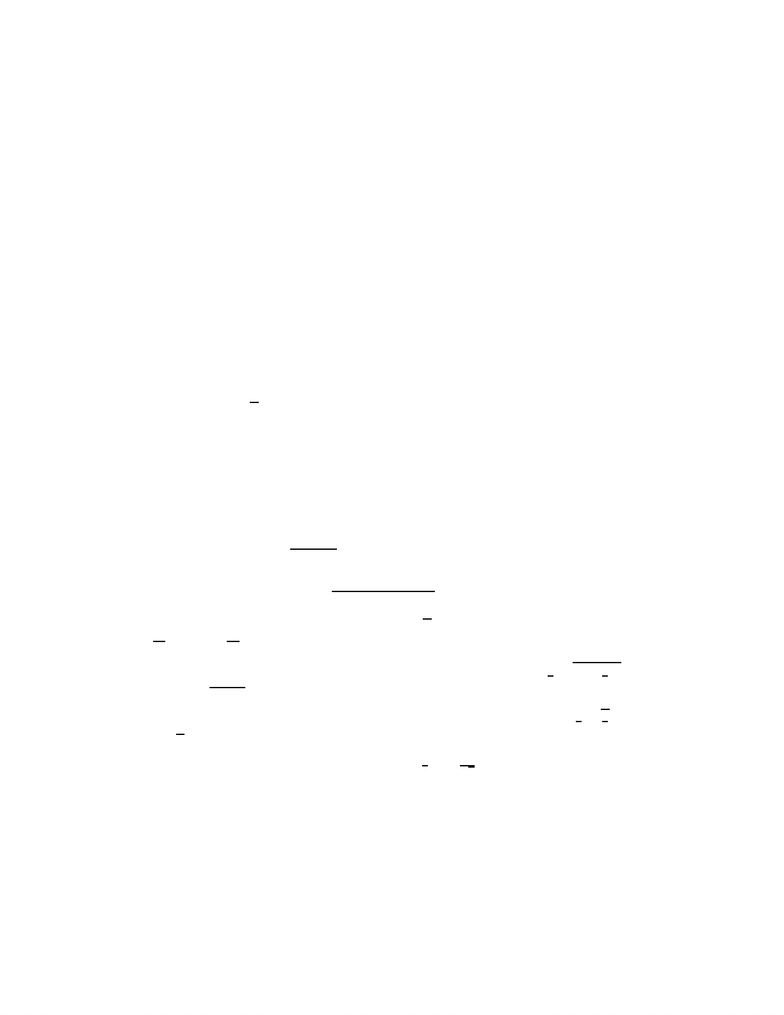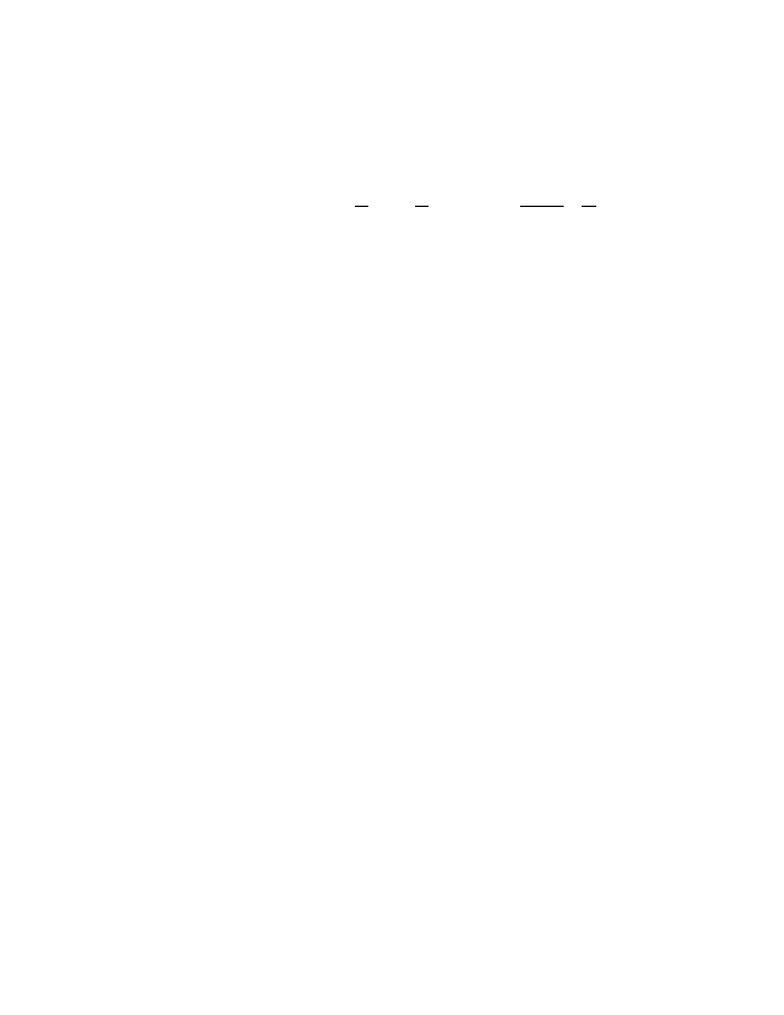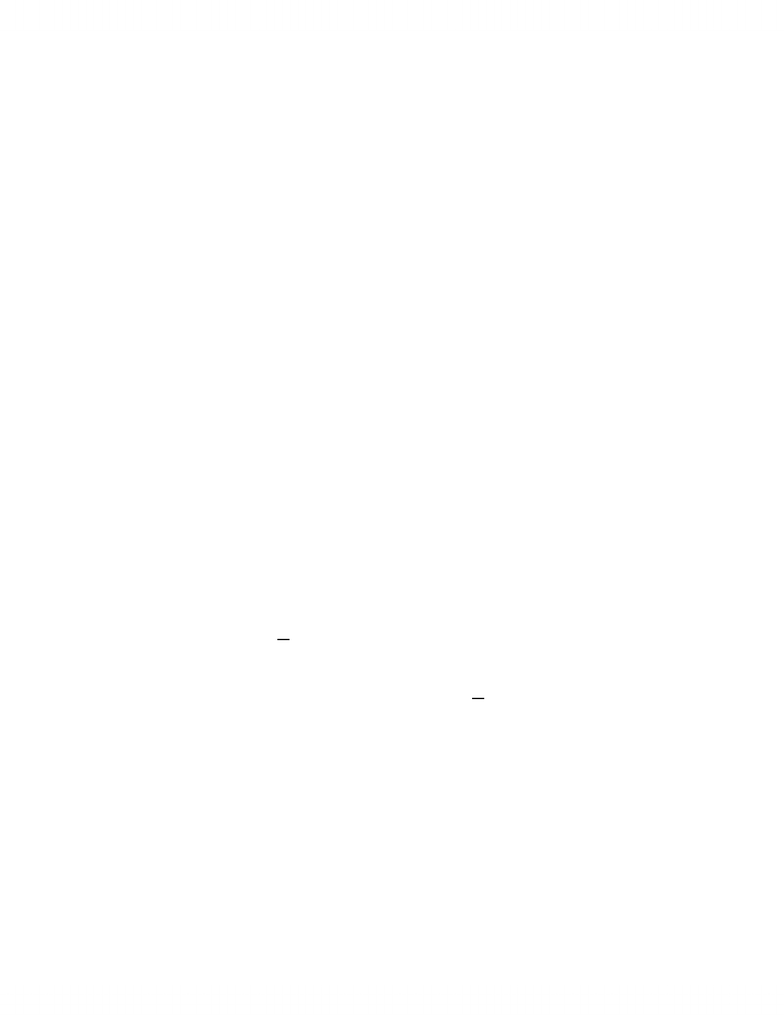# MAT237Y1 Study Guide - Riemann Integral, Level Set, Riemann Sum

35 views15 pages
School
Department
Course
ProfessorMath 237Y1
Jeremy Wong
Section L0201, 2006-07
(last updated 3/12/07)
Here are additional exercises to supplement the acknowledged paucity of problems in the textbook, roughly
grouped according to the chapter material they pertain to. Within each chapter heading, the exercises are not placed
in any particular order. The majority of the exercises are computational, rather than conceptual in nature. Starred
problems are ones which are more involved or diﬃcult, and therefore are ones which are not likely to be on a Term
Test. Additional exercises and short answers will be posted in the future.
In this handout, not all material from all chapters is represented among the types of questions below. Also,
as mentioned, some parts of this handout-in-progress (such as questions on integration) need to be updated in the
future.
1 Chapter 1
1. Show that the closure Sis connected if SRnis connected
2. True or false: If f:SRwhere SRn, and all partial derivatives jfexist and are bounded on
S, then fis continuous on S.
3. Suppose f:RmRnis a map such that for any compact set KRn, the preimage set f1(K) =
{xRm:f(x)K}is compact. Is fnecessarily continuous?
4. Show that if f:SRnis uniformly continuous and Sis bounded then f(S) is bounded.
5. Determine whether lim
(x,y)(0,0)
x4y3
x12 +y4exists, and if so, its value.
6. (*) Prove or disprove that lim
(x,y)(0,0)
xy5x3y3+ 7x6y
x5+y5exists.
7. (*) Give an example of a set SR2, a point (a, b)S, and a diﬀerentiable function f:SRsuch
that f
x (x, y) and f
y (x, y)0 as (x, y)(a, b), but for which f(x, y)→ ∞ as (x, y)(a, b).
8. (i) Let Lidenote the line segment in R2from the origin (0,0) to the point ( 1
i,q11
i2) on the unit
circle f(x) = 1x2. Is the union S:= S
i=1 Licompact?
(ii) Let Lidenote the line segment in R2from the origin (0,0) to the point ( 1
i,q1
i) on the curve
f(x) = x. Is the union S:= S
i=1 Licompact?
9. Deﬁne a sequence recursively by x1= 1 and xk+1 =1
2xk+1
k. Show that lim
k→∞xkexists and ﬁnd its
value.
2 Chapter 2
1. Find the global minimum and maximum, if any, of (x2+4xy)ey2on the set S={(x, y)R2:x21}.
2. Find and classify the critical points of
(i) f(x, y) = 3xeyx3e3y
(ii) f(x, y) = (x21)2(x2yx1)2
(iii) f(x, y) = x2y4
1
Unlock document

This preview shows pages 1-3 of the document.
Unlock all 15 pages and 3 million more documents.3. (#4, sect. 2.7) Use a Taylor series approximation to ex2to compute R1
0et2dt to three decimal
4. (*) Instead of expanding a real-valued function f(x, y) of two variables by a Taylor polynomial in
several variables, suppose one ﬁrsts expands f(x, y) as a polynomial in x, treating yas constant, so
that
f(x, y) = g0(y) + g1(y)x+g2(y)x2
2! +g3(y)x3
3! +···+gn1(y)xn1
(n1)! +xn
n!gn(x, y)
where the gi(y) (i= 1,...,n1) are functions of y, and the last term gn(x, y) possibly involves both
xand y. If n= 2 and fis C2, then is g2(x, y) necessarily C2as well?
5. Suppose fis a C2function whose Hessian matrix at some point afor which f(a) = 0is
2 0 0
03 0
0 0 7
.
Is this point a local maximum, local minimum, or neither?
6. Find and classify all possible local extrema of the function f(x, y) = (2xx2)(2yy2).
7. Suppose the post oﬃce adopts a new regulation that only boxes whose sum of length, width and height
is less than 2 meters can be mailed through their overnight delivery service. Under this regulation,
could you mail a package overnight which contained 0.3m3worth of goods?
3 Chapter 3
1. (i) Explain why the z-axis in R3cannot be represented as the level set of a C1function F:R3R.
(ii) Explain why the upper half-plane {(x, y)R2:y0}cannot be represented as a level set of a C1
function F:R2R. (It can, however, be represented a sublevel or superlevel set, e.g., F1([0,)),
where F(x, y) = y.)
2. Let f(x,y) = (x + 6y,3xy,x23y2), where x, y R. Describe the image of f.
3. Show that if F:R2Ris a continuous function and the set {(x, y)R2:F(x, y) = 0}is
disconnected, then there exists a factorization F(x, y) = G(x, y)H(x, y), for some functions Gand H.
(This is a converse to exercise #6, sect. 3.2)
4. Find the tangent plane to the surface
(i) given by f(u,v) = (u + v,4u2+ 1,uv) when u= 1, v = 2
(ii) given by F(x, y, z) = xy z2+y= 0, at (x, y, z) = (3,2,2)
5. Are the functions
f(x, y, z) = x+y2
g(x, y, z) = y+z2
h(x, y, z) = z+x2
functionally dependent on some open set UR3? If so, determine the rank of Dfat each point of U,
where f= (f,g,h).
6. (# 8, sect. 3.1) Near which points (x, y, z, u, v) = (x0, y0, z0, u0, v0), if any, can the equations
xy2+xzu +yv2= 3
u3yz + 2xv u2v2= 2
be solved for uand vas functions of x, y, z?
2
Unlock document

This preview shows pages 1-3 of the document.
Unlock all 15 pages and 3 million more documents.7. Sketch by hand the curve or surface determined by each of the following equations
(i) x3+y36xy = 0 (rectangular coordinates) in R2
(ii) r22rsin θ= 3 (cylindrical coordinates) in R3.
8. (Conversion between implicit (level set) and parametric descriptions)
(a) Find a parametric description of the intersection of the following two equations:
x2+ 5(y1)2+ 4(z+ 2)2= 1
2x+yz3 = 0.
(b) Find either a real-valued function F(x, y, z) or a vector-valued function F(x,y,z) = (F(x,y,z),G(x,y,z))
whose 0-level set is the image of the map f(u,v) = (2uv,v2,u + v).
9. (#5, sect. 3.4) Find a one-to-one C1map ffrom the ﬁrst quadrant of the x-yplane to the ﬁrst
quadrant of the u-vplane such that the region where x2y2x2and 1 xy 3 is mapped to a
rectangle. Compute the inverse map f1.
10. (i) Give a full statement of the Implicit Function Theorem and the Inverse Function Theorem.
(ii) (#7, sect. 3.4) Assuming the Inverse Function Theorem, prove the Implicit Function Theorem.
(note: it is possible to prove the Inverse Function Theorem directly, so this would not be a circular argument.)
11. Does there exist a C1map f:R2Rwhich is one-to-one? (recall that a map fis one-to-one or
injective if the images of two distinct points are distinct. Equivalently, if f(x) = f(y) then x=y.)
Hint: examine the derivative of f.
12. (*) Does there exist a C1map f:RR2which is onto? (recall that a map fis onto or surjective
if its image is the entire target space. Equivalently, the question is: can there exist a C1function f
such that f(R) = R2holds?) Hint: the notion of zero content (from chapter 4) could be helpful in
answering this. See Proposition 4.19(c), for example.
4 Chapter 4
(In problems 1-5 below, integrability refers to Riemann integrability, instead of improper Riemann integra-
bility, although several of the results do extend to the latter situation.)
1. (i) Let f(x) = (1 if x=1
2nfor some n= 0,1,2,...
0 otherwise
Is f(x) (Riemann) integrable on [0,1]?
(ii) Same question again, for the function f(x) = (1 if x=k
2nfor some kZand n= 0,1,2, . . .
0 otherwise
2. (#5, sect. 4.1) Suppose fis integrable on [a, b]. Show that |f|is integrable on [a, b] and |Rb
af(x)dx| ≤
Rb
a|f(x)|dx.
3. Does there exist a function f: [a, b]Rsuch that |f|is integrable on [a, b], but fitself is not
integrable on [a, b]?
4. Suppose f,g: [a, b]Rare integrable on [a, b]. Show that their pointwise product f·g, deﬁned as
(f·g)(x) = f(x)g(x), is integrable on [a, b].
5. If f: [a, b]Ris a function such that f2is integrable on [a, b], does it follow that fitself is integrable
on [a, b]?
6. Let CRnbe a (Jordan) measurable set. Suppose that Aand Bare disjoint subsets such that
AB=C. Prove that: Ais measurable Bis measurable.
3
Unlock document

This preview shows pages 1-3 of the document.
Unlock all 15 pages and 3 million more documents.